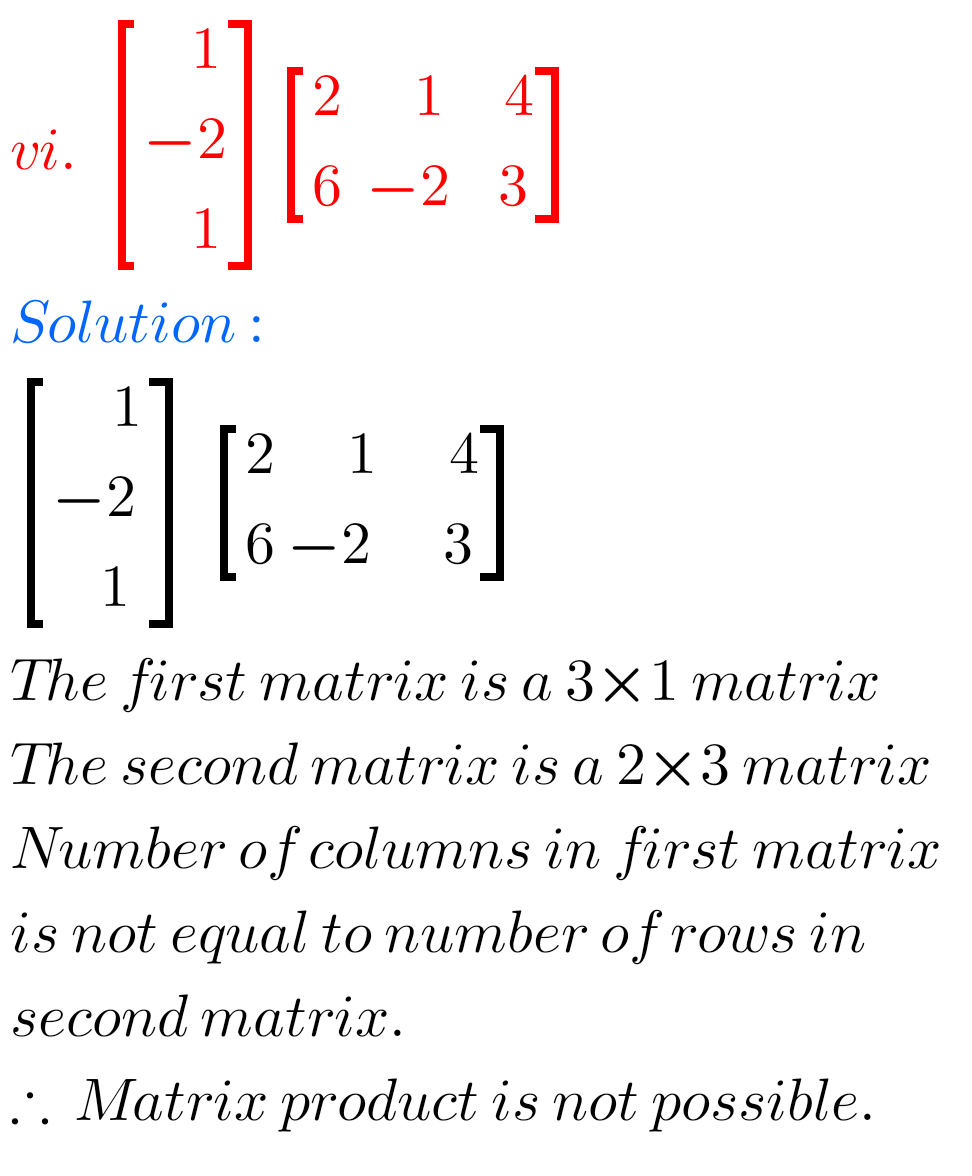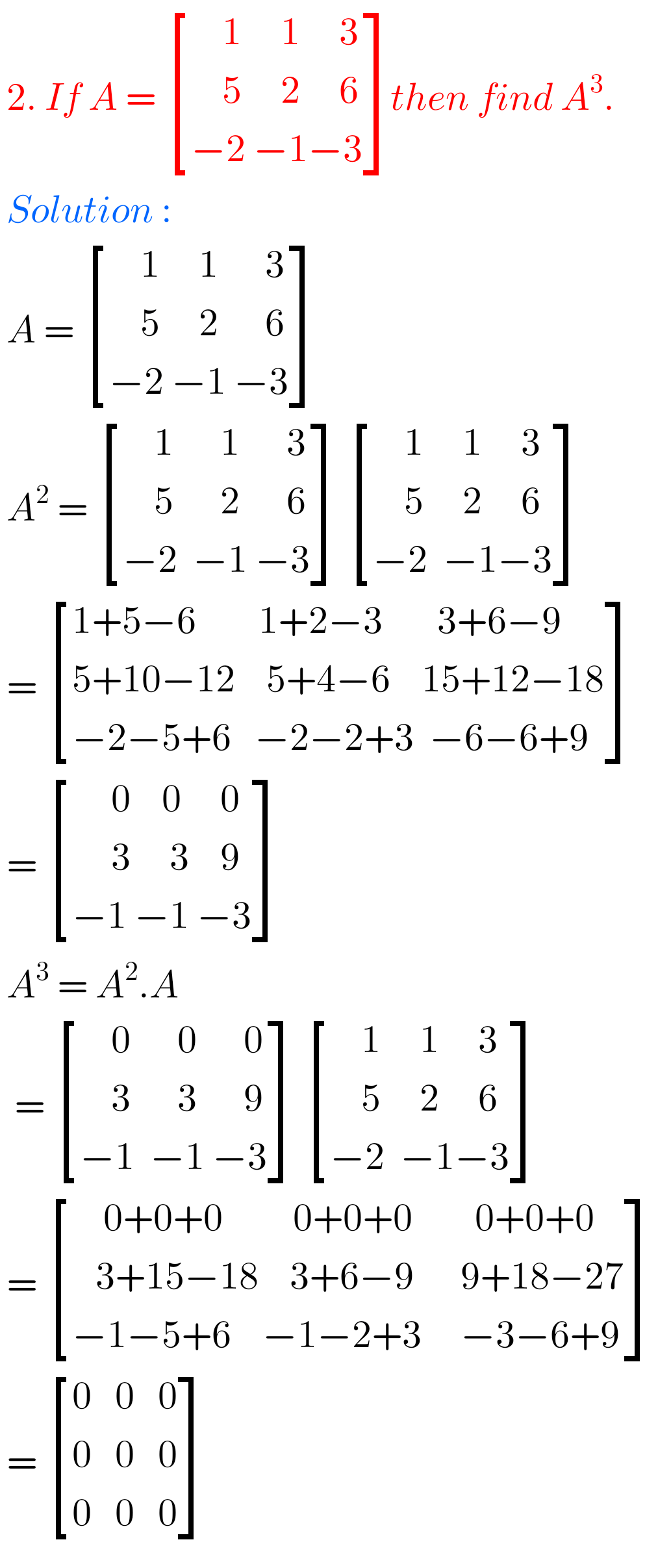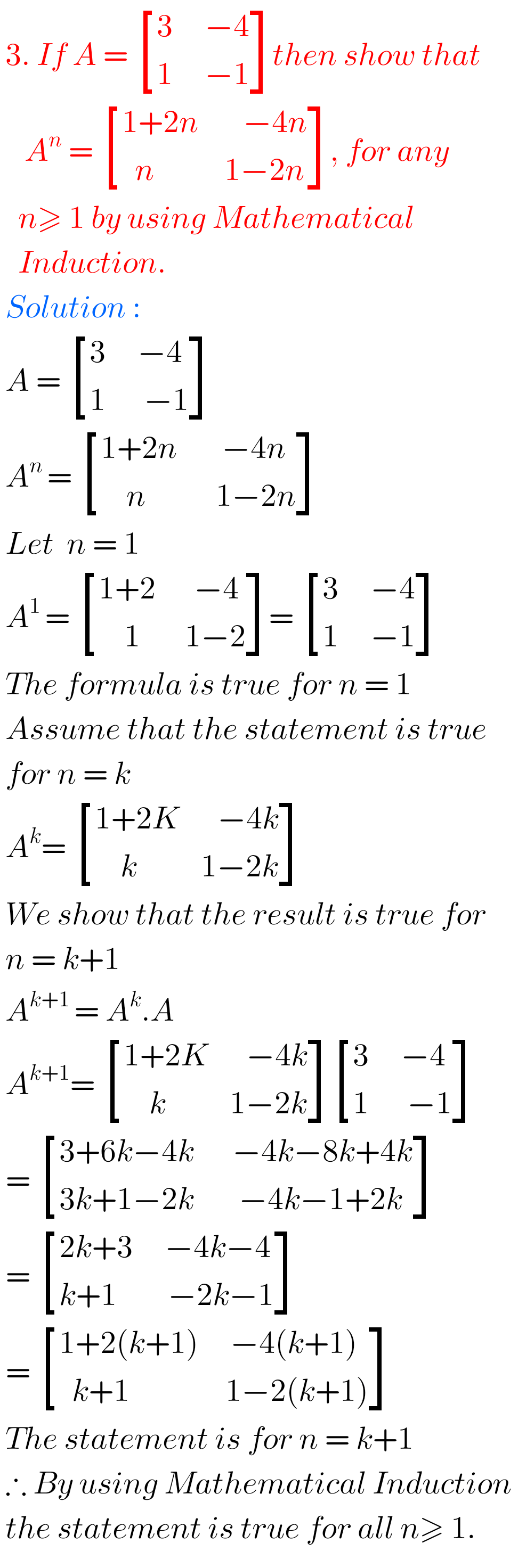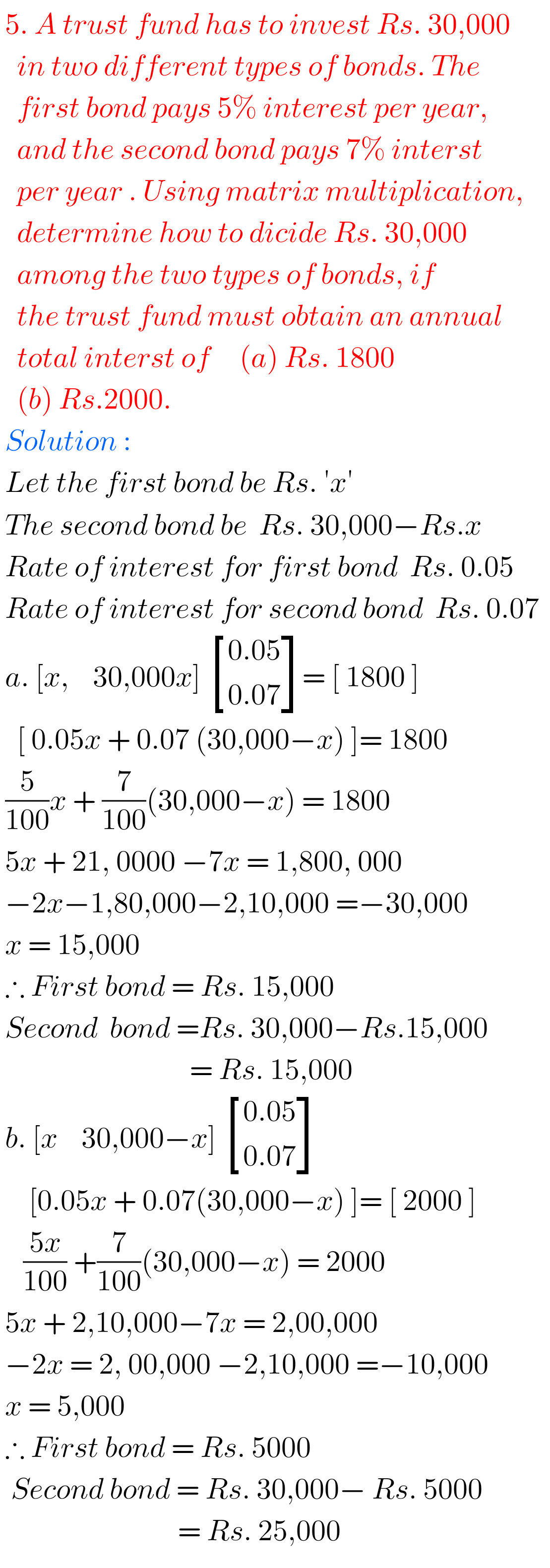# Intermediate Maths Solutions for Exercise 3(b) Matrices

Inter Maths 1A text book Exercise 3(b) Matrices solutions are given.

These solutions are very easy to understand.

Please study the text book lesson Matrices very well.

Observe the example problems and solutions given in the text book.

Observe the given solutions and try them in your own method.

You can also see

SSC Maths text book Solutions class 10

Inter Maths 1A solutions

Inter Maths 1B solutions

Inter Maths 1IA text book solutions

Inter Maths IIB text book solutions

Matrices

Exercise 3(a)

Exercise 3(b)

Exercise 3(c)

Exercise 3(d)

Exercise 3(e)

Exercise 3(f))

Exercise 3(g)

Exercise 3(h)

Exercise 3(i)

M

# Inter Maths Solutions for Matrices Exercise 3(b)

Chapter 3 Matrices

Exercise 3(b)#### Maths 1A Solutions for Matrices Exercise 3(b)## Matrices solutions exercise 3(b) InterNote :

Observe the solutions and try them in your own method.

Ncert maths class 6 chapter 14 solutions

Nios maths 311 book 1 Sets 1.1 solutions

You can see the solutions for junior inter maths 1A for examination purpose

2. Matrices